# The Most Common and Useful Features of Excel 2021

Excel is wonderful tool to work with the Data. whereas Data plays a very important role to make business rise. If you know how to work with the Data then you are aware how to work or deal with the any kind of business. Many Tech Giants like Facebook, Google, Microsoft just focuses on data and by analyzing the behavior of data they are making money like water with very less efforts. In this blog you are going to learn some basics steps and tools which can be useful for a beginner to understand or analyze the data. This blog will be containing 3 major sections which will further contain the other subsections. So lets get started…

Click here to Check out Previous Blog : Basics of ExcelHow to analyze the data data using excel

Section 1 : Checking the count of specific data.

There are 3 major used functions are available in Excel which is used for counting any row or column.

• Count(Start Address : End Address) Function : This function is used calculate the count of Numerical values like 1,2,3,4, 5….1000 up to infinity.

Lets see how to use this function :

To work with this function lets create a sample data like this: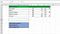Sample data to work with excel

# Count() Function :

Lets calculate the total science marks using count function because it can calculate the numerical values.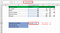How to apply count function in excel ?

After applying the formula : Count(Start Address : End Address) Function we have received the below mentioned output which is : 5 and it is correct.

# CountA() Function :

This is used to calculate the alphanumeric data so lets calculate the number of students available.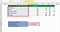how to use CountA function

By applying counta() function using same technique like we did for Count(). The only thing changed here is the function name and address which is B2:B6. The output comes as follows: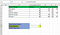Output of CountA

# CountBlank() Function

There are some empty values are available in the math column lets calculate the empty values by applying same technique. we just need to change the function name and address, for which we want to calculate the empty values.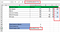count blank function

The output of the count blank function will be as expected: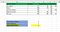Output of the count blank function

Section 2 : Data Validation

If we don't want a user to enter any impure data in our excel sheet then data validation comes into the picture. There are many types of data validations are available which are as follows: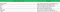Types of data validation in Excel

Let's take one example, How to implement the data validation.

# Whole number Data Validation:

whole number data validation majorly validates the data for the whole number.

Step 1: Select the column and move the cursor to data in the menu bar, follow the below image.

Step 2: choose data validation as per the below image.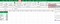Excel Validation

Step 3: choose whole in allow, and add the max and min value as shown in the below image.

Step 4: After validation, if we put a number out of the range it will throw an error as shown the below image.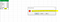error in excel

# Decimal Data Validation:

Decimal validation majorly focuses on floating values.

Follow the above step to navigate to the data validation dialog box and then change the validation as per the below image:

So this is how we do the validation if we want to enter any decimal number in our column and to avoid any mistake.

# List Data Validation:

Follow the above step to navigate to the data validation dialog box and then change the validation as per the below image:

After applying the validation we can see that a dropdown is coming on the selected cell.

# Input/ Error Message

Follow the above step to navigate to the data validation dialog box and then change the validation as per the below image:

Input Message: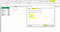input data validation

For error message follow the below image: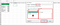error data validation

This is how we do validation, we can try with some examples in the next blog.

These are the ways using that we can implement the functions in Excel. There are several functions that can be used for many purposes Like, If, Countif, CountIfs, SumIf, SumIfs, Choose, RandBetween.

Click here to learn more about these functions.

Hope you enjoyed this small blog and the content of this blog will help you to get started with the data analysis journey in your domain. The credit of blogs to Kamaljeet Singh and wakeupcoders Team.

Click here to Download the excel used in this session.

Thank you for reading…

We let make your business smarter and broader through the power of the internet. Researcher | Web developer | Internet of things | AI | www.wakeupcoders.com

## More from Wakeupcoders

We let make your business smarter and broader through the power of the internet. Researcher | Web developer | Internet of things | AI | www.wakeupcoders.com